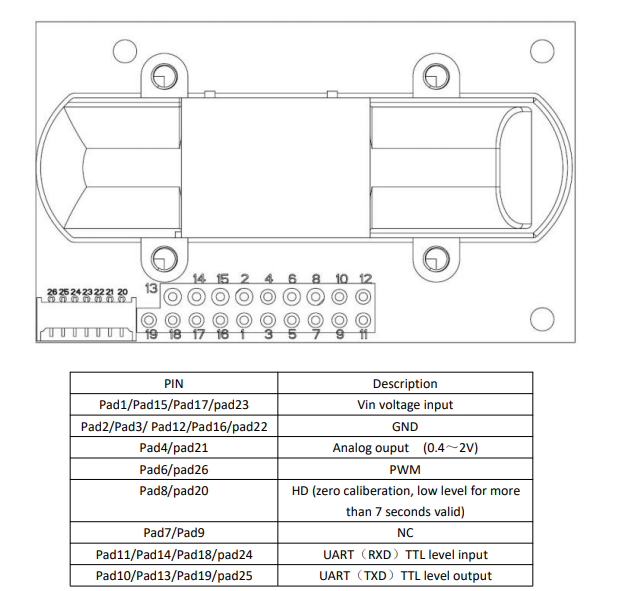# 用 Arduino 與 ESP32 打造空氣品質監測應用（2）- 資料溝通篇 UART

1. 感測器介紹篇 - DHT11 與 MH-Z14A
2. 資料溝通篇 - UART（實作是用 UART，所以只講 UART）
3. Arduino 踩雷篇
4. （未上映）WiFi 篇：為了省下 Debug 的時間，我額外購買了 ESP32 開發版，本身已經含有 WiFi 跟藍芽功能
5. （未上映）MQTT 篇：為了把資料傳給其他設備，使用了 MQTT 這個輕薄短小的通訊協定
6. （未上映）Grafana / Web 篇：資料存在資料庫當然要用炫炮的方式顯示出來啊！這裡使用了 Grafana + Promethus 以及 Svelte 來顯示資料。

• SPI
• I2C

## 傳遞資料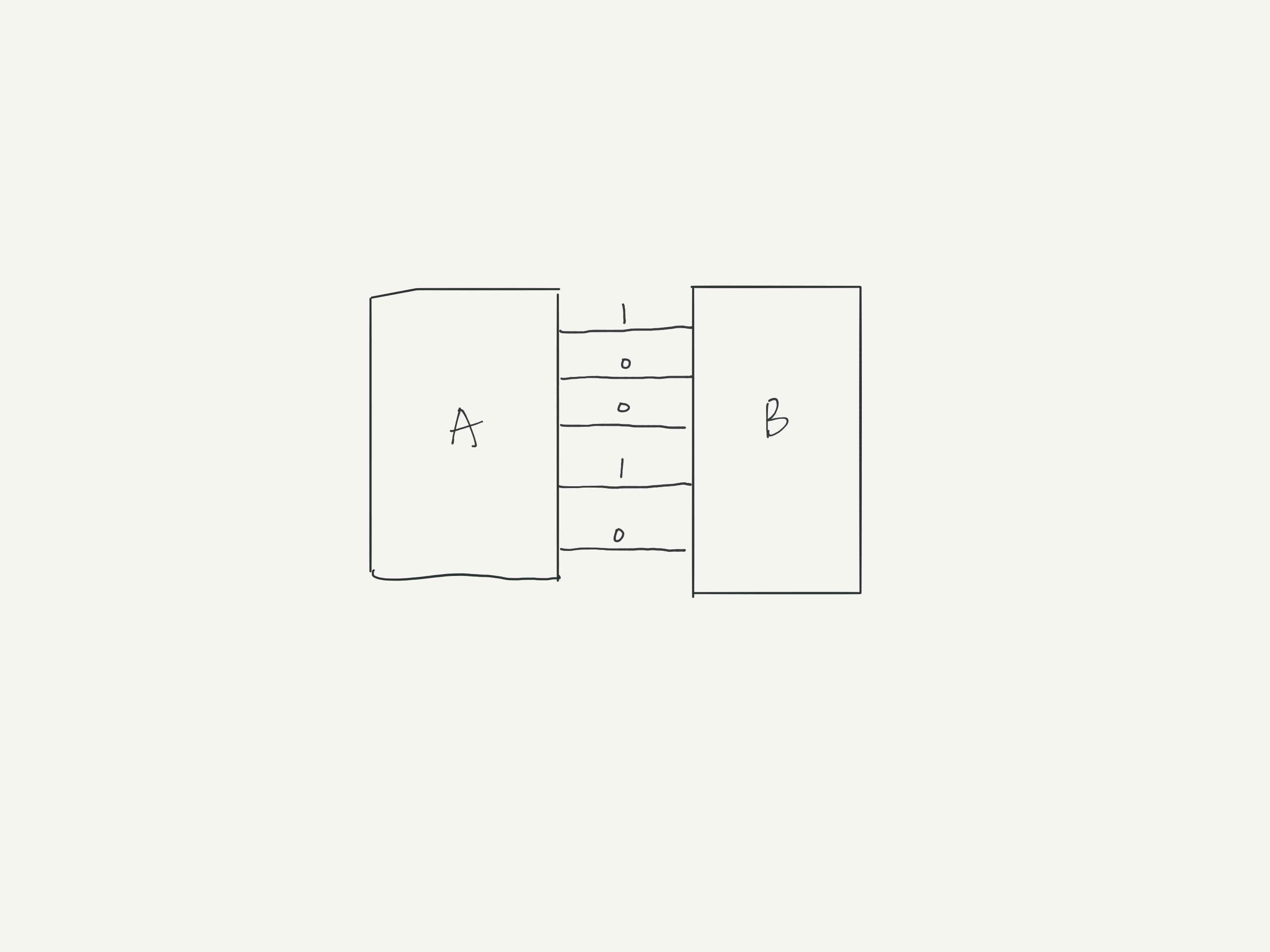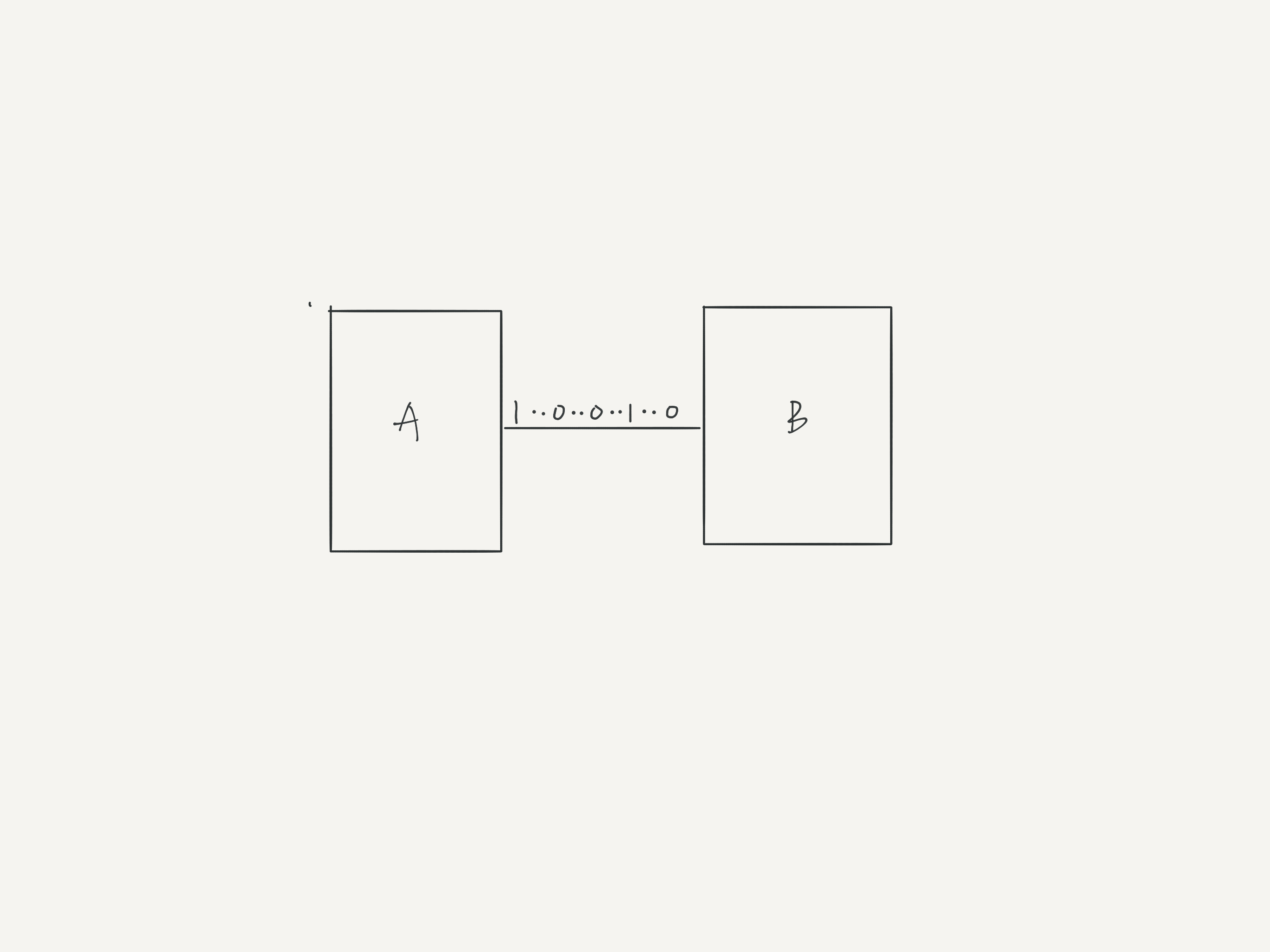## UART 資料交換

UART 中定義了 start bit 以及 stop bit 讓雙方知道何時會開始送資料、何時停止。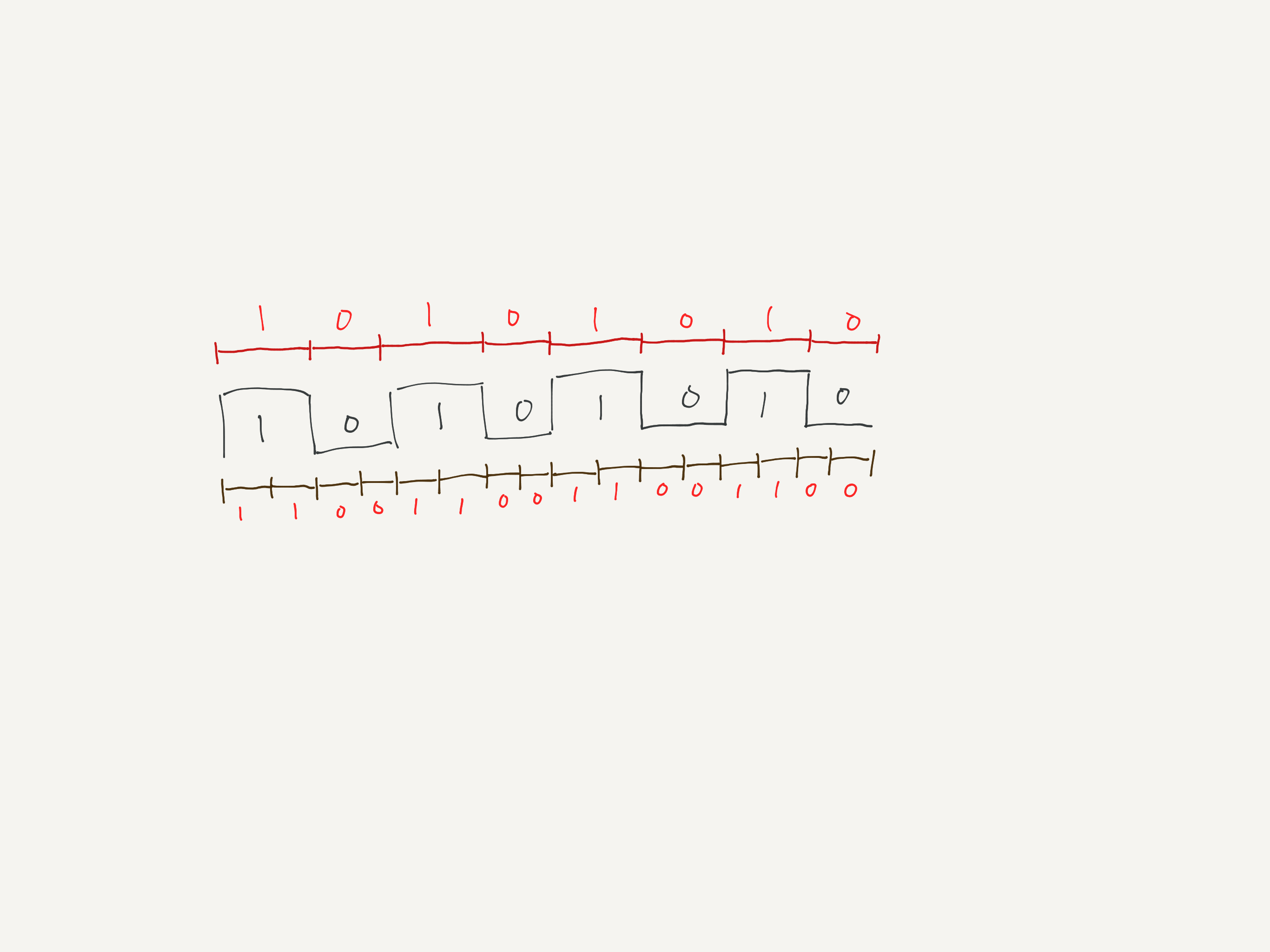• 兩個硬體設備需要共用同一個 GND
• Baud rate 必須相同

## Arduino 中的 UART

UART 是由獨立的 IC 提供或是寫在 MCU 裡頭的電路，不過 UART chip 在 Arduino 只有一個，如果有超過一個以上的設備也想要跟 Arduino 作 UART 通訊呢？或是想要將原生的 Serial Port 當作 Debug Console 方便除錯，不想給其他設備使用的話該怎麼辦？以我們的情境來說，我們想要透過 UART 跟 MH-Z14A 溝通；同時也想要透過 UART 傳送資料給 ESP32。

• 根據 datasheet 描述，這個 MH-Z14A 的感測器的傳輸率是 9600Hz。代表每個 bit 的傳送時間是 1/9600 = 1041.6666666us。首先 Arduino 的 delay 最多只能到 delayMicroSecond，所以後面的小數點必須捨棄（因為浮點數的關係，傳入 delay 的參數一定為整數），但這樣的誤差其實已經足以讓資料讀取不正確了。
• CPU 的 instruction cycle 也要花時間，這個時間雖然短，但在 UART 的通訊過程中每個 bit 累積起來的誤差也不可忽略
• 我們猜測單純使用 delay 的話，可能會被 Arduino 其他的中斷干擾導致時間不準確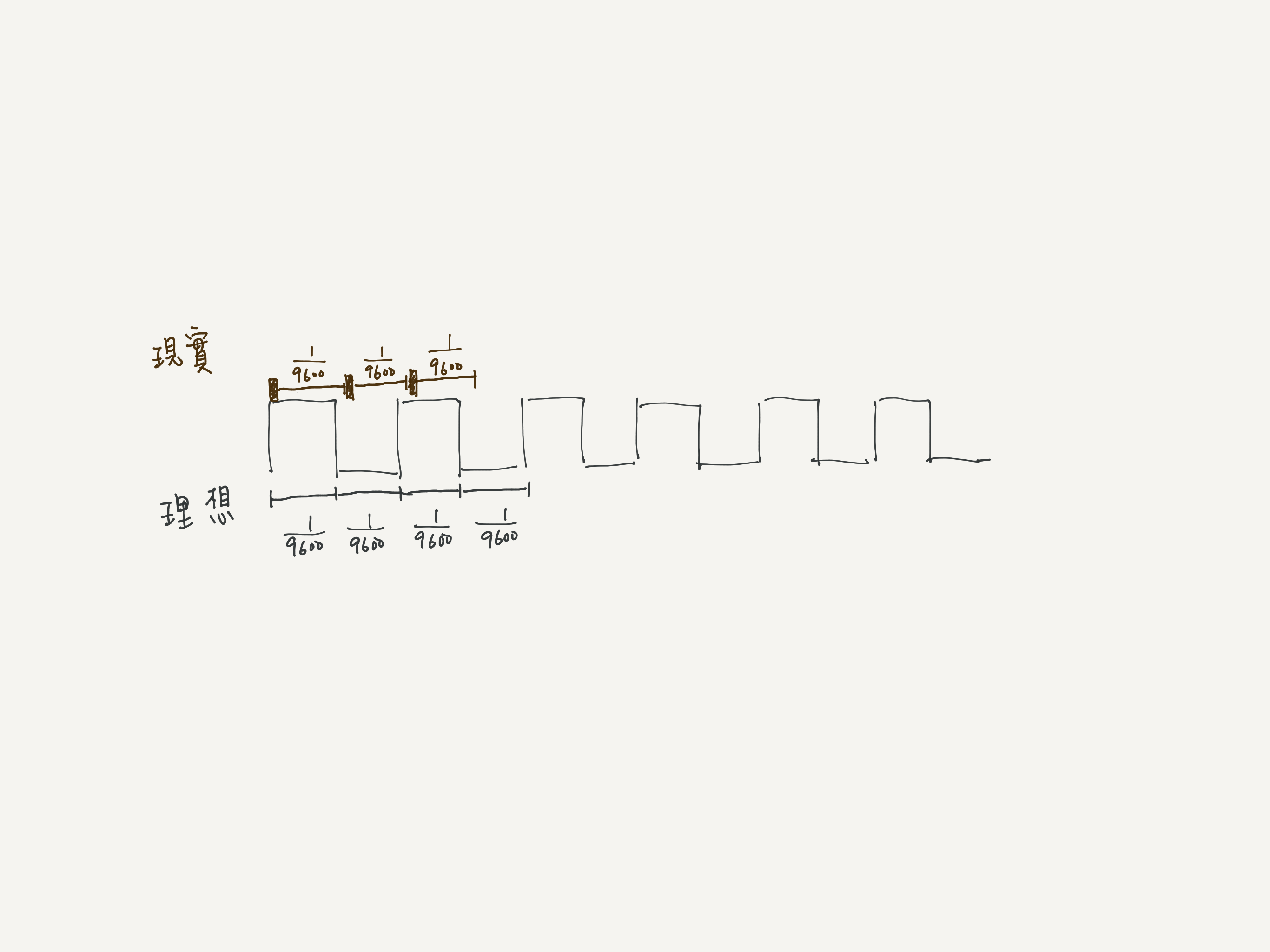MH-Z14A 的 baud rate 為 9600，每個 bit 的時間應該為 1/9600 秒，對於 CPU 來說是幾個 cycle 呢？為了方便計算我們也直接將 CPU 頻率設定為 9600Hz 好了，這樣就剛好會是 1 cycle，計算方式為：$\frac{CPU speed}{baud-rate}$

Software::begin 中可以看到實作

cvoid SoftwareSerial::begin(long speed){  // 略  // Precalculate the various delays, in number of 4-cycle delays  uint16_t bit_delay = (F_CPU / speed) / 4;  // 12 (gcc 4.8.2) or 13 (gcc 4.3.2) cycles from start bit to first bit,  // 15 (gcc 4.8.2) or 16 (gcc 4.3.2) cycles between bits,  // 12 (gcc 4.8.2) or 14 (gcc 4.3.2) cycles from last bit to stop bit  // These are all close enough to just use 15 cycles, since the inter-bit  // timings are the most critical (deviations stack 8 times)  _tx_delay = subtract_cap(bit_delay, 15 / 4);  // Only setup rx when we have a valid PCINT for this pin  if (digitalPinToPCICR((int8_t)_receivePin)) {    #if GCC_VERSION > 40800    // Timings counted from gcc 4.8.2 output. This works up to 115200 on    // 16Mhz and 57600 on 8Mhz.    //    // When the start bit occurs, there are 3 or 4 cycles before the    // interrupt flag is set, 4 cycles before the PC is set to the right    // interrupt vector address and the old PC is pushed on the stack,    // and then 75 cycles of instructions (including the RJMP in the    // ISR vector table) until the first delay. After the delay, there    // are 17 more cycles until the pin value is read (excluding the    // delay in the loop).    // We want to have a total delay of 1.5 bit time. Inside the loop,    // we already wait for 1 bit time - 23 cycles, so here we wait for    // 0.5 bit time - (71 + 18 - 22) cycles.    _rx_delay_centering = subtract_cap(bit_delay / 2, (4 + 4 + 75 + 17 - 23) / 4);    // There are 23 cycles in each loop iteration (excluding the delay)    _rx_delay_intrabit = subtract_cap(bit_delay, 23 / 4);    // There are 37 cycles from the last bit read to the start of    // stopbit delay and 11 cycles from the delay until the interrupt    // mask is enabled again (which _must_ happen during the stopbit).    // This delay aims at 3/4 of a bit time, meaning the end of the    // delay will be at 1/4th of the stopbit. This allows some extra    // time for ISR cleanup, which makes 115200 baud at 16Mhz work more    // reliably    _rx_delay_stopbit = subtract_cap(bit_delay * 3 / 4, (37 + 11) / 4);    #else // Timings counted from gcc 4.3.2 output    // Note that this code is a _lot_ slower, mostly due to bad register    // allocation choices of gcc. This works up to 57600 on 16Mhz and    // 38400 on 8Mhz.    _rx_delay_centering = subtract_cap(bit_delay / 2, (4 + 4 + 97 + 29 - 11) / 4);    _rx_delay_intrabit = subtract_cap(bit_delay, 11 / 4);    _rx_delay_stopbit = subtract_cap(bit_delay * 3 / 4, (44 + 17) / 4);    #endif    // Enable the PCINT for the entire port here, but never disable it    // (others might also need it, so we disable the interrupt by using    // the per-pin PCMSK register).    *digitalPinToPCICR((int8_t)_receivePin) |= _BV(digitalPinToPCICRbit(_receivePin));    // Precalculate the pcint mask register and value, so setRxIntMask    // can be used inside the ISR without costing too much time.    _pcint_maskreg = digitalPinToPCMSK(_receivePin);    _pcint_maskvalue = _BV(digitalPinToPCMSKbit(_receivePin));    tunedDelay(_tx_delay); // if we were low this establishes the end  }  ...}

cvoid tunedDelay(uint16_t __count){	asm volatile (		"1: sbiw %0,1" "\n\t"		"brne 1b"		: "=w" (__count)		: "0" (__count)	);}

csize_t SoftwareSerial::write(uint8_t b){  volatile uint8_t *reg = _transmitPortRegister;  uint8_t reg_mask = _transmitBitMask;  uint8_t inv_mask = ~_transmitBitMask;  uint8_t oldSREG = SREG;  bool inv = _inverse_logic;  uint16_t delay = _tx_delay;  if (inv)    b = ~b;  cli();  // turn off interrupts for a clean txmit  // Write the start bit  if (inv)    *reg |= reg_mask;  else    *reg &= inv_mask;  tunedDelay(delay);  // Write each of the 8 bits  for (uint8_t i = 8; i > 0; --i)  {    if (b & 1) // choose bit      *reg |= reg_mask; // send 1    else      *reg &= inv_mask; // send 0    tunedDelay(delay);    b >>= 1;  }  // restore pin to natural state  if (inv)    *reg &= inv_mask;  else    *reg |= reg_mask;  SREG = oldSREG; // turn interrupts back on  tunedDelay(_tx_delay);    return 1;}

• *reg|= reg_mask 程式直接用操作暫存器的方式改變高低電位，而非一般的 digitalWrite() 的寫法。我猜是為了減少 compile 後的組語造成不必要的 instruction cycle？
• cli()：解除 Arduino 的中斷機制。要做一些原子操作或時間相關的操作時可以使用，不過這也代表在資料傳遞的過程中，其他程序會暫時無法運作？令我不解的是為什麼不用官方文件中的 noInterrupts() 而是 cli()
• SREG = oldSREG：根據描述這樣可以啟動 Interrupt？為什麼不直接呼叫 interrupts() 就好？

• 直接用 delay 精確度不夠
• 為了達到精確的時間控制，需要直接計算 cycle 數
• 在通訊的過程中中斷機制會無法使用

## SoftwareSerial

SoftwareSerial 的 API 跟 HardwareSerial（也就是預先定義的 Serial）介面相同，根據上面的程式碼實作，也已經幫我們處理好 UART 的通信，所以直接使用 Serial.write 傳送指令碼就可以了。

Not all pins on the Mega and Mega 2560 support change interrupts, so only the following can be used for RX: 10, 11, 12, 13, 14, 15, 50, 51, 52, 53, A8 (62), A9 (63), A10 (64), A11 (65), A12 (66), A13 (67), A14 (68), A15 (69).

## 使用 SoftwareSerial 向 MH-Z14A 發送指令

0x86 Gas concentration / 感測二氧化碳濃度
0x87 Calibrate zero point value / 歸零校正 這個感測器有三個歸零校正方法，傳指令是其中一個
0x99 Calibrate span point value / 修改二氧化碳偵測範圍
0x79 Start/stop auto-calibration function of zero point value / 使用（或停止）自動校正功能 在出廠時，這個感測器預設會開啟自動校正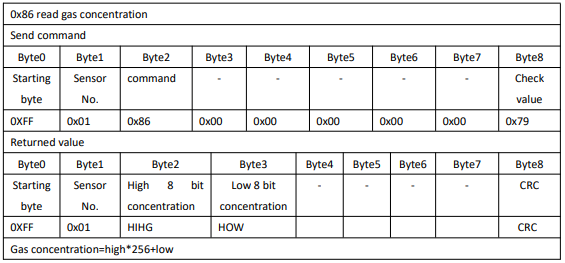cchar getCheckSum(char *packet){  char i, checksum;  for( i = 1; i < 8; i++)  {    checksum += packet[i];  }  checksum = 0xff – checksum;  checksum += 1;  return checksum;}

c#include <SoftwareSerial.h>SoftwareSerial co2Serial(3, 4); // tx, rx 接腳，只要是 digital pin 都可以void sendCommand(byte command){  byte commands = {0xff, 0x00, 0x00, 0x00, 0x00, 0x00, 0x00, 0x00, 0x00};  commands = command;  commands = getCheckSum();  co2Serial.write(commands, 9);}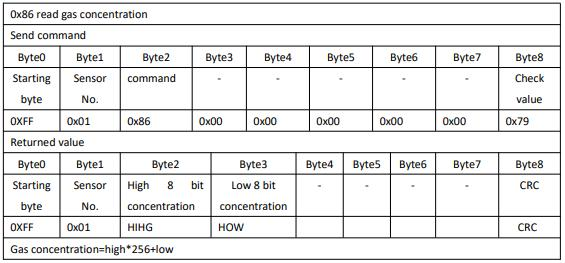cbyte responsebyte high = response;byte low = response;byte ppm = high << 8 + low;Serial.println(ppm);